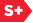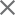### Proceedings Paper

Discrete wavelet transforms over finite sets: a general approach
Author(s): Lute Kamstra
Format Member Price Non-Member Price
PDF \$17.00 \$21.00

Paper Abstract

The discrete wavelet transform was introduced as a linear operator. It works on signals that are modeled as functions from the integers into the real or complex numbers. Since many signals have finite function values, a linear discrete wavelet transform over a finite ring has been proposed recently. Another recent development is the research of nonlinear wavelet transforms triggered by the introduction of Sweldens' lifting scheme. This paper builds on these developments and defines an essentially nonlinear translation invariant discrete wavelet transform that works on signals that are functions from the integers into any finite set. As only discrete arithmetic is needed, such transforms can be calculated very time efficiently. The basic properties of these generalized discrete wavelet transforms are given along with explicit examples.

Paper Details

Date Published: 5 December 2001
PDF: 12 pages
Proc. SPIE 4478, Wavelets: Applications in Signal and Image Processing IX, (5 December 2001); doi: 10.1117/12.449720
Show Author Affiliations
Lute Kamstra, National Research Institute for Mathematics and Computer Science (Netherlands)

Published in SPIE Proceedings Vol. 4478:
Wavelets: Applications in Signal and Image Processing IX
Andrew F. Laine; Michael A. Unser; Akram Aldroubi, Editor(s)